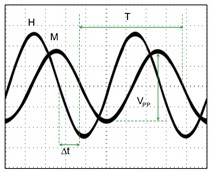# AC magnetic signal

AC magnetic signal

Under an external magnetic field, the direction of magnetic dipoles of a magnetic substance tends to be along the direction of the external magnetic field.  If the external magnetic field is an alternative-current (AC) magnetic field with low AC frequency (generally lower than microwave frequency), the magnetic dipole oscillates with this AC magnetic field.  This is the physical origin of AC magnetic susceptibility of a magnetic substance.  The oscillation frequency of magnetic dipole is the same as that of the external AC magnetic field, but a phase difference between of the magnetic dipole and the external AC magnetic field.  Therefore, the AC magnetic susceptibility χac of a material can be expressed as coeiq, where χo represents the amplitude of the magnetic susceptibility of the material, and θ is the phase difference of magnetic dipole with respect to the external magnetic field.Fig 1. The phase delay (Δt) and the amplitude (Vpp) difference between external AC magnetic field (H) and magnetic dipole (M) in certain frequency (1/T).

In addition to using magnetic susceptibility strength χo and phase difference θ to express AC magnetic susceptibility χac of a material, one can expand coeiq into the form as χocosθ+ iχosinθ.  χac can then be expressed as χr + iχi, where χr = χocosθ known as the real part of AC magnetic susceptibility (Re[Xac]), and χi = χosinθ known as the imaginary part of AC magnetic susceptibility (Im[Xac]). Therefore, the AC magnetic susceptibility χac of a material can be expressed in terms of χr and χi.Fig 2. The AC magnetic susceptibility of a magnetic substance varies with the frequency of external magnetic fields.

The relationship between χac and χdc can be written as belowTherefore, χac can be easy associated to χdc which is acquired from VSM or SQUID magnetometer.

The application of χac is not only researches for magnetic materials but also quantity measurements of magnetic materials such as magnetic fluids, materials of Li batteries, and other magnetic material, power, or thin films. The relationship between Re[Xac] and concentration, or quantity of magnetic materials is a constant under working area. The standard line can easily be drawn by simple measuring Xac at current frequency, such as below:Fig 3. The relationship between Re[cac] and the concentration of the lithium iron phosphate (LiFePO4).

The figure shows the relationship between Re[Xac] and the magetic containment of lithium iron phosphate at a certain frequency. It is clear that the Re[cac] increases linearly with the increasing concentration of magnetic containment. It is easy to determine the concentration of magnetic containment is higher or lower than 1 %.

Related Product： XacQuan, XacCryoProbe